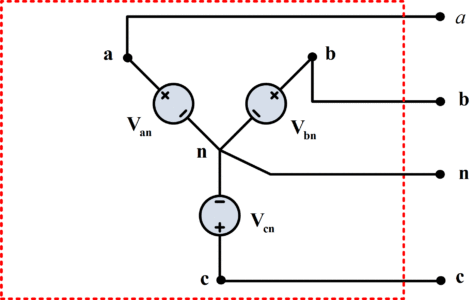# Difference between Star and Delta Connection

Want create site? Find Free WordPress Themes and plugins.

This article covers the key differences between star and delta connection on the basis of several important factors such as their configuration, voltage, current, power, motor speed, neutral point, number of turns, insulation level, and applications.

In Delta connection, phase sides are connected in a cyclical arrangement in order to make a closed loop as shown in figure 1. As far as line and phase currents are concerned, they are related to each other as:

${{I}_{line}}=\sqrt{3}{{I}_{ph}}$

Which means that whatever supply current we have, we will have wire cross-section for ${}^{1}/{}_{\sqrt{3}}$  times line current only. Whereas, in Delta connection, line and phase voltage are same:

${{V}_{line}}={{V}_{ph}}$Fig.1: Delta Connection

In star connections, fundamentally we connect the same phase sides to a mutual (common) point known as neutral point and provide supply to its free ends which stay thereafter as shown in figure 2. As far as line and phase voltages are concerned, they are related to each other as:

${{V}_{line}}=\sqrt{3}{{V}_{ph}}$

Which means that whatever supply voltage we have, we need to insulate the windings for ${}^{1}/{}_{\sqrt{3}}$  times the line voltage only. Whereas, in a Star connection, line and phase currents remain the same as:

${{I}_{line}}={{I}_{ph}}$Fig.2: Star-Connection

 Characteristics Star connection Delta connection Neutral point In star connection, there is a neutral point and it can be earthed. There is no neutral point in delta connection. Configuration In the star system, we may have two different configurations: 3 phase 3 wire system & 3 phase 4 wire system. In the delta system, only 3 phase 3 wire configuration is possible. Line voltage ${{V}_{L}}=\sqrt{3}{{V}_{ph}}$ ${{V}_{L}}={{V}_{ph}}$ Line current ${{I}_{L}}={{I}_{ph}}$ ${{I}_{L}}=\sqrt{3}{{I}_{ph}}$ Three phase power $3{{V}_{ph}}{{I}_{ph}}\cos \phi$ $3{{V}_{ph}}{{I}_{ph}}\cos \phi$ Motor speed In star configuration, motors’ speed is slow as they receive only ${}^{1}/{}_{\sqrt{3}}$ voltage. In delta configuration, motors’ speed is high as each phase gets total line voltage instead of phase voltage. Number of turns In star connection, since phase voltage is ${}^{1}/{}_{\sqrt{3}}$ times line voltage so it requires less number of turns. In the delta connection, since phase voltage is equal to line voltage so it requires more number of turns. Insulation level Low insulation is required as phase voltage is less. Heavy insulation is required as phase and line voltages are same. Applications Star configuration is primarily used in power transmission. Delta configuration is generally used in power distribution and various industrial set ups.
Did you find apk for android? You can find new Free Android Games and apps.